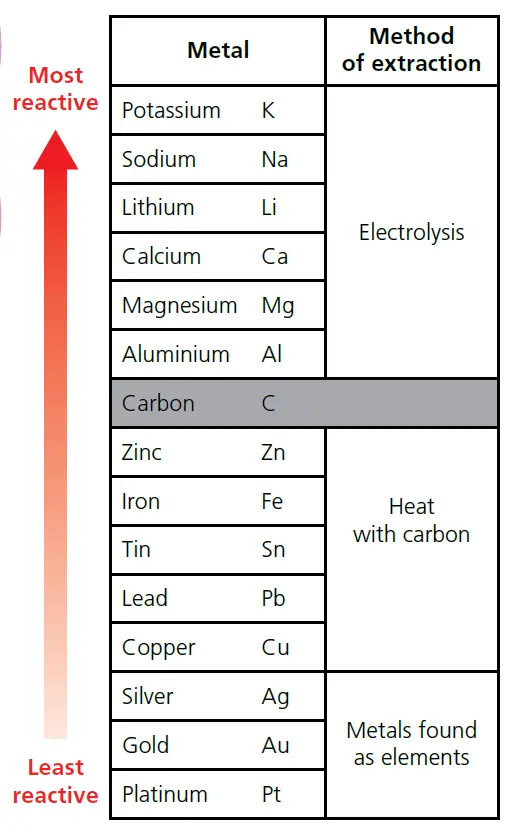# Sources of metals & methods of their extraction

In this post, we will discuss the ways of extraction of metals. In this process, we will cover ores, sources of metals, & different methods of extraction of metals (including iron extraction).

## Where do metals come from?

• A few metals, such as gold, silver and platinum, occur naturally on Earth as elements. These are metals with very low reactivity.
• Most metals are only found on Earth in compounds. For example, iron is often found in the compound iron oxide and aluminium in the compound aluminium oxide. In order to extract the metal from these compounds, a chemical reaction is required.
• The metal compounds are found in rocks called ores.
• As an example, Aluminium metal is extracted from the aluminium oxide (Al2O3) in the ore bauxite.

### What is an ore?

An ore is a rock from which a metal can be extracted for profit. As an example, Aluminium metal is extracted from the Aluminium oxide (Al2O3) in the ore bauxite.

## Methods of extraction

Most of the compounds from which metals are extracted are oxides.
In order to extract the metal, the oxygen is removed in a reduction reaction. The way in which this is done depends on the reactivity of the metal (see Figure 1 below).

• Metals that are less reactive than carbon can be extracted by heating the metal oxide with carbon. For example, iron is extracted by heating iron oxide with carbon. The iron oxide is reduced in this reaction. (figure 2)
• Metals that are more reactive than carbon can be extracted by electrolysis. For example, Aluminium, Magnesium, Calcium, Lithium, Sodium & Potassium are extracted by electrolysis.Figure 1: Extracting metals from their oxides – The way in which this is done depends on the reactivity of the metal.

## Extraction of Iron

Iron is extracted by heating iron oxide with carbon. The iron oxide is reduced in this reaction.